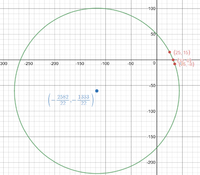# Equation of a circle through three points?

#### timburton91

##### New member
Could any one help me out how i would go about writing an equation of a circle which passes through three points?

So, if the points were (25,15 (35, -8) (32,0)?

I for the life of me can not seem to figure this out...

Any help is great, thank you#### tkhunny

##### Moderator
Staff member
Hard to say where you are going wrong, since you have not shared your efforts.

#1 Make SURE your three points are NOT collinear.

Otherwise, it's not possible.

#2a Quick estimate of Center...ummm...(25+35+32)/3 = 30.666... and (15 - 8 + 0)/3 = 2.333..., so (30.666..., 2.333...), then ask yourself if this is REALLY an estimate? It is. Don't be fooled. Make sure you know why. Since it is an estimate, how far can it be off? Ponder the radius.

#2b You may also wish simply to plot the points and eyeball an estimate of the center AND the radius.

This will empower you to know when you wander off.

#3 You need a Center (h,k) and a radius (r). Write the general equation, then plug-n-chug. No?

This may challenge your algebra skills.Worst case scenario? Quadratic expressions.

#4 Interesting woodworkers' trick. Given points A, B, and C on a circle, construct the line segment AB. Construct the line segment AC. Construct perpendicular bisector of both AB and AC. The two bisectors intersect at the center of the circle. On a piece of wood, you just need a couple of lines with a straightedge and compass and a mark with a pencil. There is a little more effort to produce the exact analytical solution.

#### MarkFL

##### Super Moderator
Staff member
Hello, and welcome to FMH!I would begin with the system:

$$\displaystyle (25-h)^2+(15-k)^2=r^2\implies h^2-50h+k^2-30k+850=r^2$$

$$\displaystyle (35-h)^2+(8+k)^2=r^2\implies h^2-70h+k^2+16k+1289=r^2$$

$$\displaystyle (32-h)^2+k^2=r^2\implies h^2-64h+k^2+1024=r^2$$

Suppose you subtract one of the equations from another...what do you get?

#### Jomo

##### Elite Member
You can do the same as the proof for showing that three non-colinear points lie on a unique circle.

Draw three non-colinear points A, B an C.

Draw a line from A to B. Then Draw the perpendicular bisector for this line. ANY point on this line can serve as the center of a circle containing the points A and B. Do you see that?

Now draw a line from B to C. Then Draw the perpendicular bisector for this line. ANY point on this line can serve as the center of a circle containing the points B and C.

The point where these two perpendicular bisectors meet will be the center of the circle containing A, B and C. Do you see that?

Can you continue from here? There is just one last step.

•hoosie

#### timburton91

##### New member
Hello, and welcome to FMH!I would begin with the system:

$$\displaystyle (25-h)^2+(15-k)^2=r^2\implies h^2-50h+k^2-30k+850=r^2$$

$$\displaystyle (35-h)^2+(8+k)^2=r^2\implies h^2-70h+k^2+16k+1289=r^2$$

$$\displaystyle (32-h)^2+k^2=r^2\implies h^2-64h+k^2 +1024=r^2$$

Suppose you subtract one of the equations from another...what do you get?
I'm with you right up untill the end portions of those equations where on earth does, 850 come from? :s

#### Subhotosh Khan

##### Super Moderator
Staff member
I'm with you right up untill the end portions of those equations where on earth does, 850 come from? :s
252 + 152 = 625 + 225 = ?

#### Jomo

##### Elite Member
Hard to say where you are going wrong, since you have not shared your efforts.

#1 Make SURE your three points are NOT collinear.

Otherwise, it's not possible.

#2a Quick estimate of Center...ummm...(25+35+32)/3 = 30.666... and (15 - 8 + 0)/3 = 2.333..., so (30.666..., 2.333...), then ask yourself if this is REALLY an estimate? It is. Don't be fooled. Make sure you know why. Since it is an estimate, how far can it be off? Ponder the radius.

#2b You may also wish simply to plot the points and eyeball an estimate of the center AND the radius.

This will empower you to know when you wander off.

#3 You need a Center (h,k) and a radius (r). Write the general equation, then plug-n-chug. No?

This may challenge your algebra skills.Worst case scenario? Quadratic expressions.

#4 Interesting woodworkers' trick. Given points A, B, and C on a circle, construct the line segment AB. Construct the line segment AC. Construct perpendicular bisector of both AB and AC. The two bisectors intersect at the center of the circle. On a piece of wood, you just need a couple of lines with a straightedge and compass and a mark with a pencil. There is a little more effort to produce the exact analytical solution.
Sorry I did not read to the end of your post where you suggested the same method I suggested a few posts later. I however prefer not to call it a woodworkers' trick but rather a proof.

•tkhunny

#### LCKurtz

##### Full Member
Using Jomo’s method I have provided an example - hope it helps!View attachment 15879
Maybe it's just me, but I think your choice of fonts is terrible. It really detracts from the readability of your work.

•Romsek, pka and MarkFL

#### MarkFL

##### Super Moderator
Staff member
Hello, and welcome to FMH!I would begin with the system:

$$\displaystyle (25-h)^2+(15-k)^2=r^2\implies h^2-50h+k^2-30k+850=r^2$$

$$\displaystyle (35-h)^2+(8+k)^2=r^2\implies h^2-70h+k^2+16k+1289=r^2$$

$$\displaystyle (32-h)^2+k^2=r^2\implies h^2-64h+k^2+1024=r^2$$

Suppose you subtract one of the equations from another...what do you get?
Using a CAS, I obtained:

$$\displaystyle (h,k,r^2) = \left(-\frac{2583}{22}, -\frac{1333}{22}, \frac{6290629}{242}\right)$$

And so the equation of the circle is:

$$\displaystyle \left(x+\frac{2583}{22}\right)^2+\left(y+\frac{1333}{22}\right)^2=\left(\frac{1}{11}\sqrt{\frac{6290629}{2}}\right)^2$$•Subhotosh Khan